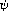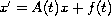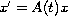Electron. J. Diff. Equ., Vol. 2010(2010), No. 102, pp. 1-9.

### Behavior at infinity of psi-evanescent solutions to linear differential equations Pham Ngoc Boi

Abstract:
In this article we present some necessary and sufficient conditions for the existence of-evanescent solution of the nonhomogeneous linear differential equation, which is related to the notion of-ordinary dichotomy for the equation. We associate that with the condition of-ordinary dichotomy for the homogeneous linear differential equation x'=A(t)x.

Submitted April 29, 2010. Published July 28, 2010.
Math Subject Classifications: 34A12, 34C11, 34D05.
Key Words: psi-bounded solutions; psi-ordinary dichotomy; psi-evanescent solutions

Show me the PDF file (222 KB), TEX file, and other files for this article.Pham Ngoc Boi Department of Mathematics, Vinh University Vinh City, Vietnam email: pnboi_vn@yahoo.com